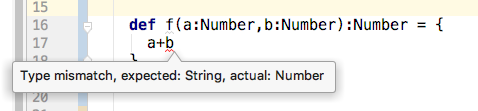# Can't add object of type Number

I don’t understand why I can’t add object whose type is `Number`.

``````    def f(a:Number,b:Number):Number = {
a+b
}
``````Is `Number` just a type inherited from Java which in the end is simply not usable? Or does Number also somehow include numbers encoded as `String`?

Number is an abstract type in Java meant to be inherited by concrete subclasses. It does not have math operators; such operators are available in various instance implementations of subclasses. Being an abstract class in java means it cannot be instantiated and thus cannot exist as an actual object by itself.

I believe `Number` isn’t what you want. Instead, I’d recommend the Numeric typeclass, which is a reasonably useful generalization of numbers. Here’s an example.

2 Likes

The Number class does not have a “+” operator (nor an “add” method). You must use one of the Number.xyzValue() methods (such as intValue(), byteValue(), etc.) to cast/convert the Number to a specific numeric value, which then you can add together

Brian Maso

And here’s an example of how to use `+` with Numeric. The extra import adds the implicits needed to provide the standard math operators…

1 Like

This Numeric[N] seems to almost work. But there does not seem to be a < defined.
see example

Well, there’s no syntax sugar for it (the sugar only exists for the basic arithmetic operations) – you have to use the operation directly on the typeclass instead. Updated example.

1 Like

OK, this looks promising… Thanks for the magic.

If you add `import num.mkOrderingOps` you can do `a < b`.

So it does.example